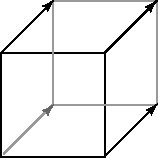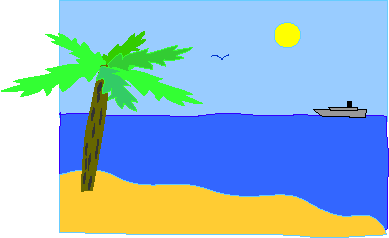A point is a location in space.

# VectorIn geometry, a point is a location in space. A point does not have any size, its only property is location. In computer graphics, a point is usually the vertex of a 3D figure.

A geometrical vector has two properties: length and direction. A vector does not have a fixed location in space. If it did, it would be a line segment. It seems odd to talk about something that does not have a location but this makes 3D computer graphics easier.

This combination of "distance and direction" is sometimes called a displacement. Often the same displacement (i.e. just one displacement) is applied to each of several points. For example, consider a cube. The front face contains four vertices (four points). If you move the same distance and direction from each of these points you reach the four vertices of the back face.

The "same distance and direction" is a vector, shown here as a line with an arrow head. The diagram shows this one vector four times, once for each point of the face.

### QUESTION 5:Say that it is Spring Break and you are at the beach and the Sun is shining down. Is the light from the Sun shining down in the same direction for everyone on the beach?